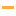Math Games

Play the best free online Math Games! Check out the Top 100 Math games from PrimaryGames.

#41Two Minute Warning: Multiplication Flashcards - By 1Learn multiplication facts and how to multiply by 1 in this online flashcard game. You have 2 minutes to solve as many multiplication problems as you can. Use the keyboard or touchscreen to play and become a times table expert.

#42Turkey Fives
#43Squigly's Apples
#44Math Mosaics: HalloweenIt's color by number! To determine the color of each space solve each addition problem, then use the code at the side of the page. The final picture will be revealed as each square is filled.

#45Grand Prix Racing: MultiplicationRace against other online players while practicing your multiplication skills this fun math game.

#46Math Tiles: Christmas MultiplicationPractice multiplication skills with factors up to 12 in this fun puzzle game.

#47Mathcopter 2Try to fly through all five levels while flying into balloons containing the fractions that are equal to the fraction at the bottom of the screen.

#48Comparing NumbersPractice comparing two numbers between 1 and 10 in this fun online math game. The alligators are hungry! They have a huge appetite and always want to eat the bigger number. Practice saying if one number is less than, greater than, or equal to another...

#49Math Mosaics: ChristmasIt's color by number! To determine the color of each space solve each addition problem, then use the code at the side of the page. The final picture will be revealed as each square is filled.

#50Spring Math MunchmanPractice addition, subtraction, and multiplication in this math game. Gobble up power pellets showing the correct answer to the math problem shown. Don't eat the wrong answer or you will lose a life.

#51Christmas Count Link PuzzlePractice counting skills by matching the number of objects shown in each picture to the correct number. Complete all of the questions to reveal a Christmas picture.

#52Math Mosaics: SpringIt's color by number! To determine the color of each space solve each addition problem, then use the code at the side of the page. The final picture will be revealed as each square is filled.

#53Math Eggs: Even & Odd NumbersCatch eggs from even or odd numbered chickens according to the label on the basket. The score will be decreased if you catch the wrong numbers. You have 3 lives. Watch out! You will lose a life when an egg with the correct number breaks on the ground.

#54Math Tiles: Groundhog Day Addition and SubtractionPractice addition and subtraction skills in this fun puzzle game. Select from adding, subtracting or both and choose between a range of 10, 20 or 100.

#55Easter Egg Patterns
#56Math Riddles: WinterLook at each picture math equation. Use the information from equation to solve for the question mark. What number should replace the question mark?

#57Math Riddles: EasterLook at each picture math equation. Use the information from equation to solve for the question mark. What number should replace the question mark?

#58Math Tiles: St. Patrick's Day MultiplicationPractice multiplication skills with factors up to 12 in this fun puzzle game.

#59Kitty Add 123
#60Two Minute Warning: Multiplication Flashcards - By 10Learn multiplication facts and how to multiply by 10 in this online flashcard game. You have 2 minutes to solve as many multiplication problems as you can. Use the keyboard or touchscreen to play and become a times table expert.